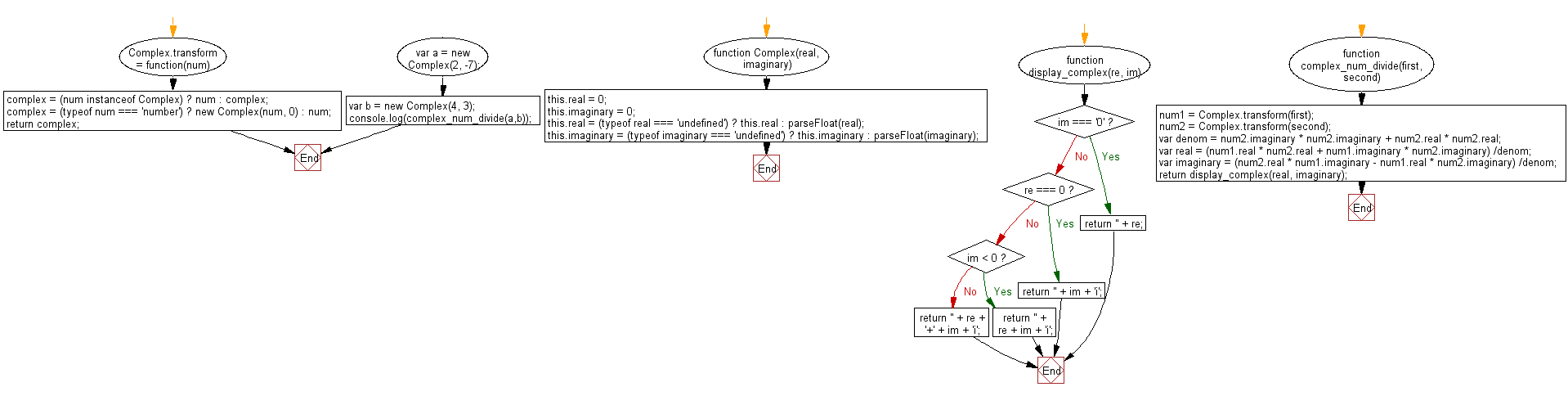# JavaScript: Divide two complex numbers

## JavaScript Math: Exercise-53 with Solution

Write a JavaScript program to divide two complex numbers.
A complex number is a number that can be expressed in the form a + bi, where a and b are real numbers and i is the imaginary unit, that satisfies the equation i2 = −1. In this expression, a is the real part and b is the imaginary part of the complex number.

Sample Solution:-

HTML Code:

``````<!DOCTYPE html>
<html>
<meta charset="utf-8">
<title>JavaScript function to divide two complex numbers</title>
<body>

</body>
</html>
```
```

JavaScript Code:

``````function Complex(real, imaginary) {
this.real = 0;
this.imaginary = 0;
this.real = (typeof real === 'undefined') ? this.real : parseFloat(real);
this.imaginary = (typeof imaginary === 'undefined') ? this.imaginary : parseFloat(imaginary);
}
Complex.transform = function(num) {
var complex;
complex = (num instanceof Complex) ? num : complex;
complex = (typeof num === 'number') ? new Complex(num, 0) : num;
return complex;
};
function display_complex(re, im) {
if(im === '0') return '' + re;
if(re === 0) return '' + im + 'i';
if(im < 0) return '' + re + im + 'i';
return '' + re + '+' + im + 'i';
}
function complex_num_divide(first, second) {
var num1, num2;
num1 = Complex.transform(first);
num2 = Complex.transform(second);
var denom = num2.imaginary * num2.imaginary + num2.real * num2.real;
var real = (num1.real * num2.real + num1.imaginary * num2.imaginary) /denom;
var imaginary = (num2.real * num1.imaginary - num1.real * num2.imaginary) /denom;
return display_complex(real, imaginary);
}
var a = new Complex(2, -7);
var b = new Complex(4,  3);
console.log(complex_num_divide(a,b));
```
```

Sample Output:

```-0.52-1.36i
```

Flowchart:Live Demo:

See the Pen javascript-math-exercise-53 by w3resource (@w3resource) on CodePen.

Improve this sample solution and post your code through Disqus

What is the difficulty level of this exercise?

Test your Programming skills with w3resource's quiz.

﻿